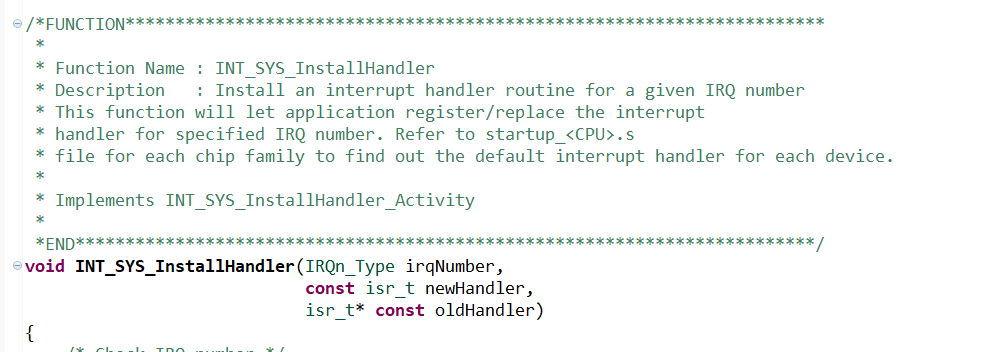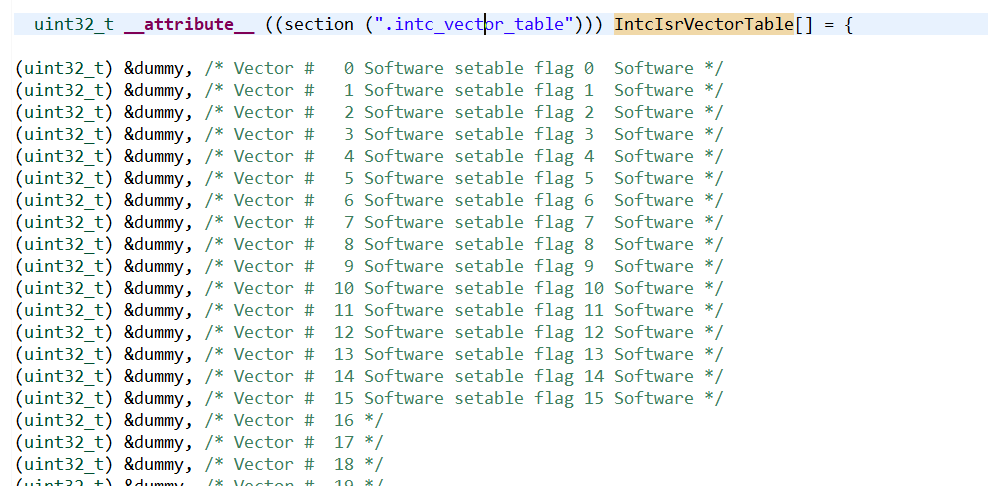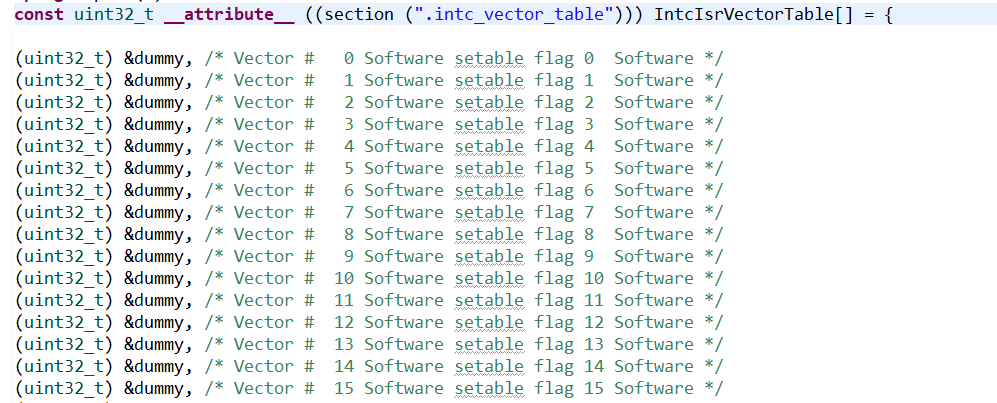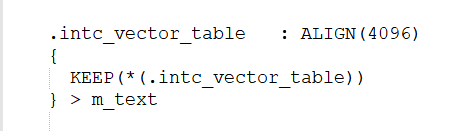# How can I change the vector table?

cancel
Showing results for
Did you mean:
SOLVED

## How can I change the vector table?

2,746 ViewsContributor II

Hello!

I have a new problem about the interrupt.

First,I can entry the IRQ function by following the demo and it's correctly.

But now,I want to set a function like this:I have tried,but I failed.

So,my problem is that I just set the IRQ funtion before compiling in this array:but I want to get a function to install the IRQ funtion.How can I do?

Tags (2)
1 Solution
2,358 ViewsNXP Employee

Hi,

normally - the vector table is copied in the RAM - to be able change IRQ handler address in runtime. As you can see from startup - the vector table is defined at beginning of flash (m_text) and in startup is copied to RAM (0x40000000) and IACKR0->VTBA is set to 0x40000 (aligned to 0x1000) in startup.c.

So, if you create another vector table (in the FLASH area) with your function (and there is still present the default vector table) - you also need to modify startup.c where vector table is copied from FLASH to RAM:

if (__VECTOR_RAM != __VECTOR_TABLE)
{
/* Copy the vector table from ROM to RAM */
for (n = 0; n < (((uint32_t)__RAM_VECTOR_TABLE_SIZE)/sizeof(uint32_t)); n++)
{
__VECTOR_RAM[n] = __VECTOR_TABLE[n];
}
/* Point the VTOR to the position of vector table */
*vectors[coreId] = (uint32_t)__VECTOR_RAM;
}
else
{
/* Point the VTOR to the position of vector table */
*vectors[coreId] = (uint32_t)__VECTOR_TABLE;
}

Hope it helps.

Jiri

4 Replies
2,358 ViewsNXP Employee

Hi,

If I'm understand it correctly - you like to add Interrupt handler in compile time, correct?

So - just add your particular handler instead &dummy - that's all. Don't forget declare this handler like the dummy is and of course you need to implement it.

For example:

void dummy   (void);

void MyPitHandler(void);

(uint32_t) &dummy, /* Vector # 223 */
(uint32_t) &dummy, /* Vector # 224 Real Time Counter (RTC) RTC */
(uint32_t) &dummy, /* Vector # 225 Autonomous Periodic Interrupt (API) API */
(uint32_t) &MyPitHandler, /* Vector # 226 Periodic Interrupt Timer (PIT0) PIT_1_TFLG0[TIF] */
(uint32_t) &dummy, /* Vector # 227 Periodic Interrupt Timer (PIT1) PIT_1_TFLG1[TIF] */
(uint32_t) &dummy, /* Vector # 228 Periodic Interrupt Timer (PIT2) PIT_1_TFLG2[TIF] */

Jiri

2,358 ViewsContributor II

I am sorry that i don't state my problem clearly.

Your method is a good way to solve the problem,Jiri.

But I want to ask you for another.

In fact, the array is definitionedthe array is definitioned in text section,So I can't change the value of the definitioned vector table.

Now I want to set a new arrary to store the ISRs' address,like this:

uint32_t IntcIsrVectorTable = {(uint32_t)(&dummy),(uint32_t)(&dummy) ...};

and then

INTC.IACKR.R = (uint32_t)&IntcIsrVectorTable;

last I replace the corresponding value of the array with ISR address,like:

void Pit0Ch0Isr(void)

{

PIT_0.TIMER.TFLG.B.TIF = 1;                                                                                    //clear the flag
SIUL2.GPDO.B.PDO_4n3 = ~SIUL2.GPDO.B.PDO_4n3;                                //turn

}

IntcIsrVectorTable = (uint32_t)(&Pit0Ch0Isr);

and then I enable the interrupt,I find that I can't enter the changed ISR,what happen?

2,359 ViewsNXP Employee

Hi,

normally - the vector table is copied in the RAM - to be able change IRQ handler address in runtime. As you can see from startup - the vector table is defined at beginning of flash (m_text) and in startup is copied to RAM (0x40000000) and IACKR0->VTBA is set to 0x40000 (aligned to 0x1000) in startup.c.

So, if you create another vector table (in the FLASH area) with your function (and there is still present the default vector table) - you also need to modify startup.c where vector table is copied from FLASH to RAM:

if (__VECTOR_RAM != __VECTOR_TABLE)
{
/* Copy the vector table from ROM to RAM */
for (n = 0; n < (((uint32_t)__RAM_VECTOR_TABLE_SIZE)/sizeof(uint32_t)); n++)
{
__VECTOR_RAM[n] = __VECTOR_TABLE[n];
}
/* Point the VTOR to the position of vector table */
*vectors[coreId] = (uint32_t)__VECTOR_RAM;
}
else
{
/* Point the VTOR to the position of vector table */
*vectors[coreId] = (uint32_t)__VECTOR_TABLE;
}

Hope it helps.

Jiri

2,357 ViewsContributor II

Thank you,Jiri!

According to your method,I find it is a problem that interrupt redirect,and I solved the problem already,thank you!